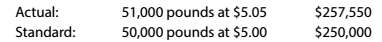Chapter 13, Problem 4SEQ

Chapter
Section
Textbook Problem

The actual and standard direct materials costs for producing a specified quantity of product are as follows:The direct materials price variance is: A. $50 unfavorable B.$2,500 unfavorable C. $2,550 unfavorable D.$7,550 unfavorable

To determine

Concept Introduction:

Direct material variances:

Direct material variances refer to the difference between the standard direct material cost and actual direct material cost incurred. Direct material cost variances are categorized into following two categories:

1. Direct material Rate variance: this variance shows the difference of standard rate and actual rate of material. The formula to calculate this variance is as follows:
2.   Direct material rate variance = (Actual rate – Standard rate) ×Actual Quantity

3. Direct material usage variance: this variance shows the difference of standard usage and actual usage of material. The formula to calculate this variance is as follows:
4.   Direct material usage variance = (Actual Quantity – Standard Quantity)×Standard rate

5. Direct material cost variance: this variance shows the difference of standard cost and actual cost of material. The formula to calculate this variance is as follows:
6.   Direct material cost variance = Direct material rate variance + Direct material usage variance

To Calculate:

The Direct Material Price variance

Explanation

The Direct Material Price variance is calculated as follows:

 Direct Material Price Variance: Actual Price (A) $5.05 Per Pound Standard Price (B)$ 5...

Still sussing out bartleby?

Check out a sample textbook solution.

See a sample solution

The Solution to Your Study Problems

Bartleby provides explanations to thousands of textbook problems written by our experts, many with advanced degrees!

Get Started

Find more solutions based on key concepts